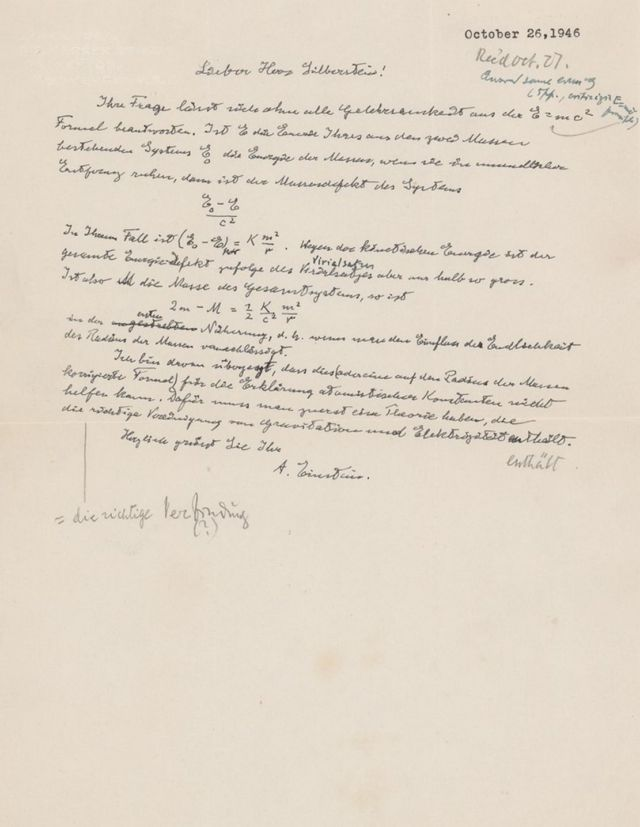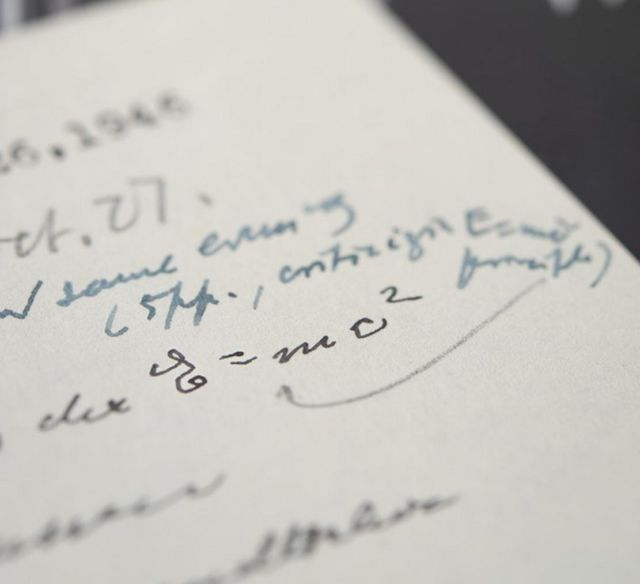Albert Einstein‘s handwritten famous Mass Equation ‘E = mc²’, sold for a whopping \$1.2 million at auction.

The rare Albert Einstein’s letter that was written to physicist Ludwik Silberstein in October 1946, sold for more than three times what was expected.

The winning bidder of the E = mc² letter, has preferred to remain anonymous.Mass–energy relation: E = mc²

In physics, mass–energy equivalence is the relationship between mass and energy in a system’s rest frame, where the two values differ only by a constant and the units of measurement. The principle is described by the physicist Albert Einstein’s famous formula.

The formula defines the energy E of a particle in its rest frame as the product of mass (m) with the speed of light squared (c²). Because the speed of light is a large number in everyday units (approximately 3×108 meters per second), the formula implies that a small amount of rest mass corresponds to an enormous amount of energy, which is independent of the composition of the matter.

Images credit RR Auctions